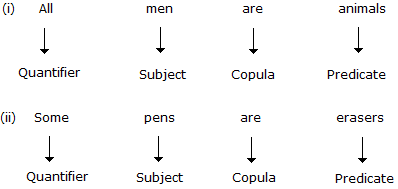# Logical Reasoning - Logical Deduction

Exercise : Logical Deduction - Introduction
Directions to Solve

In each question below are given two statements followed by two conclusions numbered I and II. You have to take the given two statements to be true even if they seem to be at variance from commonly known facts. Read the conclusion and then decide which of the given conclusions logically follows from the two given statements, disregarding commonly known facts.

• (A) If only conclusion I follows
• (B) If only conclusion II follows
• (C) If either I or II follows
• (D) If neither I nor II follows and
• (E) If both I and II follow.

In Logic, any categorical statement is termed as the Proposition.

A Proposition (or a categorical statement) is a statement that asserts that either a part of, or the whole of, one set of objects - the set identified by the subject term in the sentence expressing that statement - either is included in, or is excluded from, another set - the set identified by the predicate term in that sentence.

The standard form of a proposition is :

Quantifier + Subject + Copula + Predicate

Thus, the proposition consists of four parts :

1. Quantifier: The words 'all', 'no' and 'some' are called quantifiers because they specify a quantity 'All' and 'no' are universal quantifiers because they refer to every object in a certain set, while the quantifier 'some' is a particular quantifier because it refers to at least one existing object in a certain set.

2. Subject (denoted by 'S'): The subject is that about which something is said.

3. Predicate (denoted by 'P'): The predicate is the part of the proposition denoting that which is affirmed or denied about the subject.

4. Copula : The copula is that part of the proposition which denotes the relation between the subject and the predicate.

Examples:Four-Fold Classification of Propositions :

A proposition is said to have a universal quantity if it begins with a universal quantifier, and a particular quantity if it begins with a particular quantifier. Besides, propositions which assert something about the inclusion of the whole or a part of one set in the other are said to have affirmative quality, while those which deny the inclusion of the whole or a part of one set in the other are said to have a negative quality. Also, a term is distributed in a proposition if it refers to all members of the set of objects denoted by that term. Otherwise, it is said to be undistributed. Based on the above facts, propositions can be classified into four types :

1. Universal Affirmative Proposition (denoted by A): It distributes only the subject i.e. the predicate is not interchangeable with the subject while maintaining the validity of the proposition.

e.g., All snakes are reptiles. This is proposition A since we cannot say 'All reptiles are snakes'.

2. Universal Negative Proposition (denoted by E): It distributes both the subject and the predicate i.e. an entire class of predicate term is denied to the entire class of the subject term, as in the proposition.

e.g., No boy is intelligent.

3.Particular Affirmative Proposition (denoted by I): It distributes neither the subject nor the predicate.

e.g.,Some men are foolish. Here, the subject term 'men' is used not for all but only for some men and similarly the predicate term 'foolish' is affirmed for a part of subject class. So, both are undistributed.

4. Particular Negative Proposition (denoted by O): It distributes only the predicate. e.g., Some animals are not wild. Here, the subject term 'animals' is used only for a part of its class and hence is undistributed while the predicate term 'wild' is denied in entirety to the subject term and hence is distributed. These facts can be summarized as follows :

Statement FormQuantityQualityDistributed
(A): All S is P.UniversalAffirmativeS only
(E): No S is P.UniversalNegativeBoth S and P
(I): Some S is P.ParticularAffirmativeNeither S nor P
(O): Some S is not PParticularNegativeP only